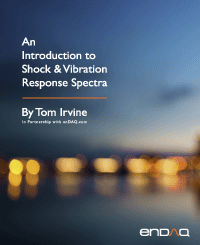Vibration Response Spectrum
By Tom Irvine
SECTION 1
VRS Basics

The vibration response spectrum overall level is calculated using equation (16.23) by varying the natural frequency for a given Q value. It can be plotted in terms of the overall 1-sigma, 3-sigma, and peak values as a function of natural frequency. The GRMS and 1-sigma values are taken to be equal because the mean is assumed to be zero. The expected peak value, or crest factor, is calculated from equation (13.15) and is the only of three response metrics that depends on duration.

The vibration response spectrum is demonstrated in the following example. First calculate the SDOF response to the Navmat P-9492 PSD base input for natural frequencies at 100, 200, and 300 Hz, with Q=10, using the model in Figure 12.12.Figure 1.1. SDOF Response to Navmat P-9492 Base Input PSD for Three Natural Frequencies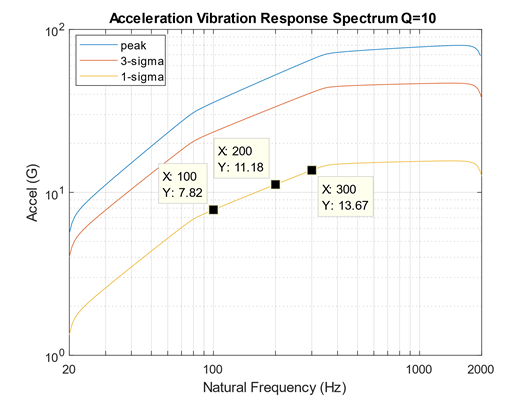Figure 1.2. Vibration Response Spectrum of Navmat P-9492 Base Input PSD

The calculation of the response PSDs in Figure 1.1 could be repeated for a larger set of natural frequencies. This process can be automated via a software program. The resulting VRS is shown in Figure 1.2.

The Figure 1.1 legend’s three coordinates are shown explicitly in the plot. A duration of 180 seconds is assumed, but this only affects the peak curve. The peak curve shows that peaks up to 4 and 5-sigma may occur over the duration.

SECTION 2
VRS Comparison of Two PSDs

The following example is actual case history. A launch vehicle avionics component was tested to the red curve in the above figure. The blue curve represents the flight data from an accelerometer mounted adjacent to the component. The flight data has three peaks that exceeded the test level. Was the component under-tested? The natural frequency of the component is unknown but could have been at any of the three exceedance peaks. Assume that each curve represents the same duration for simplicity.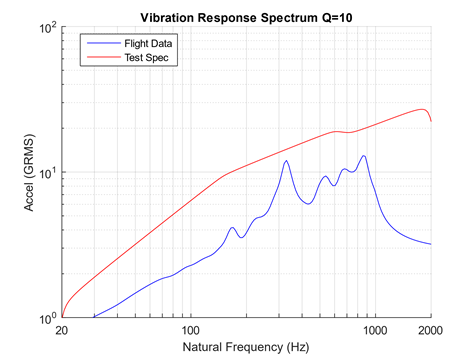Figure 2.2. Vibration Response Spectrum Comparison

The test level was 8.6 dB higher that the flight data in terms of overall level. The vibration response spectrum comparison shows that the component would have a higher response to the test level than to the flight level across the entire natural frequency spectrum from 20 to 2000 Hz. The component test level enveloped the flight data with margin to spare.

SECTION 3
PSD Envelope Derivation via VRS

The vibration response spectrum can also be used an indirect method for deriving a PSD envelope for a measured environment. The PSD envelope will have a few coordinates in log-log format as a simplification. The goal is to derive the least PSD possible that will still envelop the measured PSD in terms of the respective VRS curves. This allows for some peak clipping as demonstrated in the following example for an actual case history. There are some industry rules-of-thumb for peak clipping, but the VRS is a more thorough approach.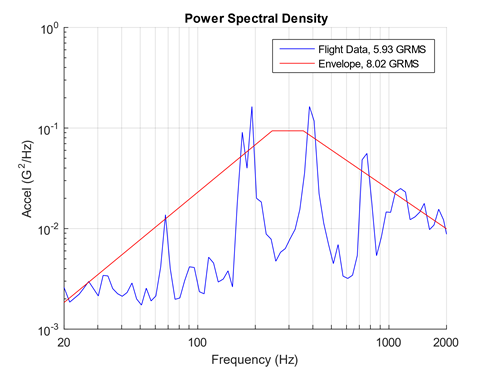Figure 3.1. Suborbital Launch Vehicle Flight PSD and Envelope

The flight data from a launch vehicle internal bulkhead location is shown in the above figure. The spectral peaks were unexpected. A realization was later made that a small access cover had been prematurely jettisoned at liftoff. The result was that cylindrical module housing the bulkhead became a large whistle. This was an anomaly which could be corrected for the next flight in this vehicle series. Nevertheless, it presented an opportunity to develop the enveloping method. The following example proceeds assuming the whistle condition would be repeated. The proposed envelope is shown with the flight data in Figure 1.5. The VRS justification for the envelope is given in Figure 1.6. Assume the flight and envelope duration are the same for simplicity. The envelope derivation process uses trial-and-error with random number generation, along with some convergence routines.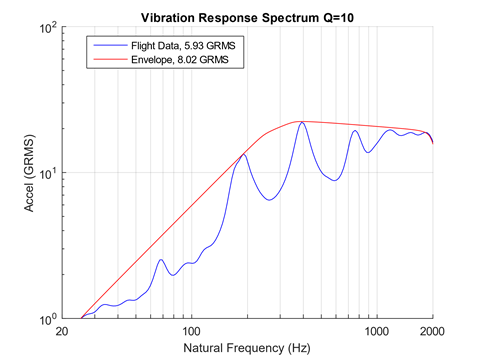Figure 3.2. Vibration Response Spectrum ComparisonFREE RESOURCE: Download Tom's Entire 287 pg Handbook - PDF